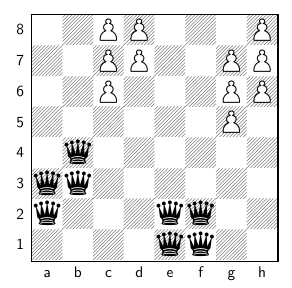Monday, December 10, 2018

More queens problems (2)

In , I discussed the Minimum Dominating Set of Queens Problem. Here is a variant:

Given an $$n \times n$$ chess board, place $$n$$ queens on the board such that the number of squares not under attack is maximized.

For an $$n=8$$ problem, the maximum number of non-dominated squares is 11. An example is:11 white pawns are not under attack

We base the model on the one in . First we define two sets of binary variables:

\begin{align} & x_{i,j} = \begin{cases} 1 & \text{if square $$(i,j)$$ is occupied by a queen}\\ 0 & \text{otherwise}\end{cases} \\ & y_{i,j} = \begin{cases} 1 & \text{if square $$(i,j)$$ is under attack} \\ 0 & \text{otherwise}\end{cases}\end{align}

The model can look like:

Maximum Non-Dominated Squares Problem
\begin{align}\max& \>\color{DarkRed}z=\sum_{i,j} \left(1-\color{DarkRed} y_{i,j}\right)\\ & \sum_{j'} \color{DarkRed}x_{i,j'} + \sum_{i'} \color{DarkRed}x_{i',j} + \sum_{i',j'|i'-j'=i-j} \color{DarkRed}x_{i',j'} +\sum_{i',j'|i'+j'=i+j} \color{DarkRed}x_{i',j'}-3 \color{DarkRed} x_{i,j} \ge \color{DarkBlue}M \cdot \color{DarkRed}y_{i,j} && \forall i,j \\ & \sum_{i,j} \color{DarkRed} x_{i,j} = n \\& \color{DarkRed}x_{i,j},\color{DarkRed}y_{i,j}\in\{0,1\} \end{align}

The funny term $$-3x_{i,j}$$ is to compensate for double counting occurrences of $$x_{i,j}$$ in the previous terms . We need to find a reasonable value for $$M$$. An upper bound is $$M=n$$. For $$n=8$$, there are 48 different optimal solutions.

The problem $$n=9$$ has one structural optimal solution (with 18 unattacked positions), and 3 variants by symmetry:

Is there a good way to find only structural solutions? One way is to use your own cuts as in , If we want to use the solution pool technology, we need to invent a constraint that forbids some symmetry (or have an objective that favors some of symmetric versions).

References

1. More queens problems, https://yetanothermathprogrammingconsultant.blogspot.com/2018/12/more-queens-problems.html
2. Bernard Lemaire, Pavel Vitushinkiy, Placing $$n$$ non-dominating queens on the $$n\times n$$ Chessboard, 2011, https://www.ffjm.org/upload/fichiers/N_NON_DOMINATING_QUEENS.pdf
3. Maximum Queens Chess Problem, https://www.gams.com/latest/gamslib_ml/queens.103